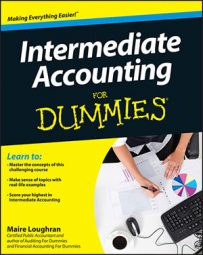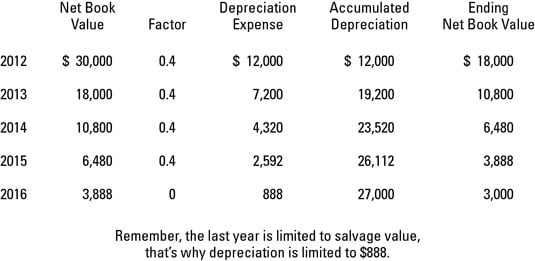##### Intermediate Accounting For DummiesDepreciation is the method of allocating costs to the appropriate period. Although accountants have to follow generally accepted accounting principles (GAAP) for financial statement reporting purposes, they have different allowable methods to consider.

Depreciation isn’t part of the whole equation for figuring the fair market value, which is the amount of money the company may fetch when it sells any of the assets.

Your intermediate accounting textbook discusses a few different methods of depreciation. Three are based on time: straight-line, declining-balance, and sum-of-the-years’ digits. The last, units-of-production, is based on actual physical usage of the fixed asset. Here’s a brief explanation of each:

• Straight-line: This method spreads the cost of the fixed asset evenly over its useful life.

• Declining-balance: An accelerated method of depreciation, it results in higher depreciation expense in the earlier years of ownership.

• Sum-of-the-years’ digits: Compute depreciation expense by adding all years of the fixed asset’s expected useful life and factoring in which year you are currently in, as compared to the total number of years.

• Units-of-production: The total estimated number of units the fixed asset will produce over its expected useful life, as compared to the number of units produced in the current accounting period, is used to calculate depreciation expense.

Psssst — just a quick word on two special methods: group and composite. Using one depreciation rate across the board, a company uses the group method when the assets are similar and have a similar expected life. And, companies use the composite method when assets are dissimilar and have different lives. These methods aren’t allowed for tax and are used in only very special circumstances for GAAP.

Generally, International Financial Reporting Standards (IFRS) and GAAP account for deprecation similarly. International Accounting Standard (IAS) 16 states that companies must review the method of depreciation periodically; if the previous estimate changes, the method must also be changed.

## Walk the straight-line depreciation method

When using the straight-line method, the salvage value reduces the depreciable base. Assume that Penway purchases a toaster fabrication machine for \$30,000. Salvage value is \$3,000. The cost of the machine (\$30,000) minus its salvage value (\$3,000) gives you a depreciable base of \$27,000.

The expected useful life is 5 years. So depreciation expense for the toaster machine is \$27,000 / 5, or \$5,400 depreciation expense per year for each of the five years. Book value at the end of year 5 is the salvage value of \$3,000.

## The declining-balance depreciation method

Don’t deduct salvage value when figuring the depreciable base for the declining balance method. But do limit depreciation so that, at the end of the day, the asset’s net book value is the same as its estimated salvage value.

You compute cost and salvage value for the asset the same as with the straight-line method. For your rate, you use a multiple of the straight-line rate.

Going back to the Penway example, the straight-line rate is 20 percent. Well, because the toaster-making machine has a useful life of five years, shown as a percentage, the straight-line rate is 1/5, or 20 percent per year.

To use the double declining-balance method shown in the figure, the multiplier is 2, so the double-declining rate is 40 percent (20% x 2).## The units-of-production depreciation method

Units-of-production is an activity method because you compute depreciation on actual physical use, making it a fantastic method for computing factory machinery depreciation.

In addition to knowing the cost basis and estimating the salvage value, you need to estimate how many outer shells the machine can produce prior to retirement. The best estimate for Mr. Toaster-Maker is 60,000 units. Taking cost minus salvage value gives you \$27,000. Divide \$27,000 by your anticipated usage of 60,000 units (\$27,000 / 60,000), which equals \$0.45. This is your unit-of-production depreciation rate.

To compute depreciation expense year after year, you multiply the actual number of units the machine makes during the year by the depreciation rate. In 2012, this is 10,100 units, so depreciation expense is \$4,545 (10,100 x \$0.45). For 2013 through 2016, the same calculation applies.

Now work through one more year. In 2013, actual units are 15,300. Depreciation is \$6,885 (15,300 x \$0.45). Accumulated depreciation is \$11,430 (\$4,545 + \$6,885) and book value is \$18,570 (\$30,000 – \$11,430).

## Calculate sum-of-the-years' digits

With this method, you come up with a depreciation fraction using the number of years of useful life. Penway’s machine has a useful life of five years. Add (5 + 4 + 3 + 2 + 1 = 15) to get your denominator for the rate fraction. In year 1, your multiplier is 5/15 (1/3); in year 2, the multiplier is 4/15; and so on.

Again, you subtract the estimated salvage value from the cost (\$30,000 – \$3,000 = \$27,000). The first year, the depreciation expense is \$9,000 (\$27,000 / 3). In the second year, the depreciation expense is \$7,200 ([\$27,000 x 4] / 15). For year 3, the depreciation expense is \$5,400 ([\$27,000 x 3] / 15).

Year 4 is \$3,600 ([\$27,000 x 2] / 15). Year 5 is \$1,800 (\$27,000 / 15).

Checking the math, you know you can’t depreciate past salvage value, so adding all five years of depreciation expense equals \$27,000. Yippee, 100 percent correct!